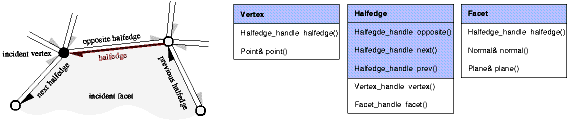## CGAL::Polyhedron_3<Traits>::Halfedge

### Definition

Figure 23.1 depicts the relationship between a halfedge and its incident halfedges, vertices, and facets. A halfedge is an oriented edge between two vertices. It is always paired with a halfedge pointing in the opposite direction. The opposite() member function returns this halfedge of opposite orientation. If a halfedge is incident to a facet the next() member function points to the successor halfedge around this facet. For border edges the next() member function points to the successor halfedge along the hole. For more than two border edges at a vertex, the next halfedge along a hole is not uniquely defined, but a consistent assignment of the next halfedge will be maintained in the data structure. An invariant is that successive assignments of the form h = h->next() cycle counterclockwise around the facet (or hole) and traverse all halfedges incident to this facet (or hole). A similar invariant is that successive assignments of the form h = h->next()->opposite() cycle clockwise around the vertex and traverse all halfedges incident to this vertex. Two circulators are provided for these circular orders.Figure 23.1:  The three classes Vertex, Halfedge, and Facet of the polyhedral surface. Member functions with shaded background are mandatory. The others are optionally supported.

The incidences encoded in opposite() and next() are available for each instantiation of polyhedral surfaces. The other incidences are optionally available as indicated with type tags. The prev() member function points to the preceding halfedge around the same facet. It is always available, though it might perform a search around the facet using the next() member function to find the previous halfedge if the underlying halfedge data structure does not provide an efficient prev() member function for halfedges. Handles to the incident vertex and facet are optionally stored.

The circulators are assignable to the Halfedge_handle. The circulators are bidirectional if the halfedge provided to the polyhedron with the Items template argument provides a member function prev(), otherwise they are of the forward category.

#include <CGAL/Polyhedron_3.h>

### Types

 Polyhedron_3::Halfedge::Vertex type of incident vertices. Polyhedron_3::Halfedge::Facet type of incident facets. Polyhedron_3::Halfedge::Vertex_handle handle to vertex. Polyhedron_3::Halfedge::Halfedge_handle handle to halfedge. Polyhedron_3::Halfedge::Facet_handle handle to facet. Polyhedron_3::Halfedge::Halfedge_around_vertex_circulator circulator of halfedges around a vertex. Polyhedron_3::Halfedge::Halfedge_around_facet_circulator circulator of halfedges around a facet. Polyhedron_3::Halfedge::Vertex_const_handle Polyhedron_3::Halfedge::Halfedge_const_handle Polyhedron_3::Halfedge::Facet_const_handle Polyhedron_3::Halfedge::Halfedge_around_vertex_const_circulator Polyhedron_3::Halfedge::Halfedge_around_facet_const_circulator Polyhedron_3::Halfedge::Supports_halfedge_prev ≡ CGAL::Tag_true or CGAL::Tag_false. Polyhedron_3::Halfedge::Supports_halfedge_vertex ≡ CGAL::Tag_true or CGAL::Tag_false. Polyhedron_3::Halfedge::Supports_halfedge_face ≡ CGAL::Tag_true or CGAL::Tag_false.

### Creation

 Polyhedron_3::Halfedge h; default constructor.

### Operations

 Halfedge_handle h.opposite () Halfedge_const_handle h.opposite () const the opposite halfedge. Halfedge_handle h.next () Halfedge_const_handle h.next () const the next halfedge around the facet. Halfedge_handle h.prev () Halfedge_const_handle h.prev () const the previous halfedge around the facet. Halfedge_handle h.next_on_vertex () Halfedge_const_handle h.next_on_vertex () const the next halfedge around the vertex (clockwise). Is equal to h.next()->opposite(). Halfedge_handle h.prev_on_vertex () Halfedge_const_handle h.prev_on_vertex () const the previous halfedge around the vertex (counterclockwise). Is equal to h.opposite()->prev(). bool h.is_border () const is true if h is a border halfedge. bool h.is_border_edge () const is true if h or h.opposite() is a border halfedge. Halfedge_around_vertex_circulator h.vertex_begin () Halfedge_around_vertex_const_circulator h.vertex_begin () const circulator of halfedges around the vertex (clockwise). Halfedge_around_facet_circulator h.facet_begin () Halfedge_around_facet_const_circulator h.facet_begin () const circulator of halfedges around the facet (counterclockwise). std::size_t h.vertex_degree () const the degree of the incident vertex, i.e., number of edges emanating from this vertex. bool h.is_bivalent () const returns true if the incident vertex has exactly two incident edges. bool h.is_trivalent () const returns true if the incident vertex has exactly three incident edges. std::size_t h.facet_degree () const the degree of the incident facet, i.e., number of edges on the boundary of this facet. bool h.is_triangle () const returns true if the incident facet is a triangle. bool h.is_quad () const returns true if the incident facet is a quadrilateral.

### Operations available if Supports_halfedge_vertex ≡ CGAL::Tag_true

 Vertex_handle h.vertex () Vertex_const_handle h.vertex () const the incident vertex of h.

### Operations available if Supports_halfedge_facet ≡ CGAL::Tag_true

 Facet_handle h.facet () Facet_const_handle h.facet () const the incident facet of h. If h is a border halfedge the result is default construction of the handle.